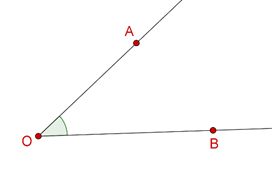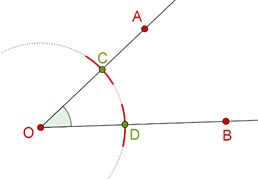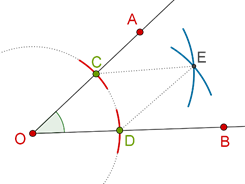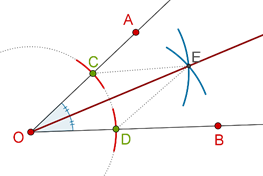# Constructing Angle Bisectors

Go back to  'Triangles-Quadrilaterals'

Consider $$\angle AOB$$ as shown below:You are asked to construct the angle bisector of this angle. How will you proceed? Note that you have not been asked for any angle measurements. You have been asked to construct the bisector, which means that you do not need a protractor. This point is important to understand. For example, if you are thinking – “Why cannot I just measure $$\angle AOB$$ with a protractor, then draw exactly half of that angle?” – you are wrong, because when no angle measurements have been asked for, we must avoid using a protractor, and use only a straight-edge and a compass. This challenge is a fundamental idea behind geometrical constructions: What kind of constructions can be accomplished with just a straight-edge and a compass?

The steps in the construction of the required angle bisector are outlined below:

Step 1: Draw an arc with center O and any radius, to cut rays OA and OB at C and D.Note that OC = OD, since these are radii of the same circle.

Step 2: Draw two arcs, with C and D as centers, and the same radius, so that these two arcs intersect at E:Note that for the two arcs to intersect, the radius you have taken in drawing these two arcs must be greater than half of CD. Can you see why?

Also, note that CE = DE, since the two arcs you drew in this step were of the same radius.

Step 3: Complete the ray OE. This is your angle bisector:It is important to understand the reason (the proof) behind why this construction works.

Proof: Compare $$\Delta OCE$$ and $$\Delta ODE$$:

1. OC = OD (radii of the same circular arc)

2. CE = DE (arcs of equal radii)

3. OE = OE (common)

By the SSS criterion, the two triangles are congruent, which means that

$$\angle COE$$ = $$\angle DOE$$

Thus, ray OE is the angle bisector of $$\angle COD$$, or $$\angle AOB$$. Note how no angle measurements were required for this construction.